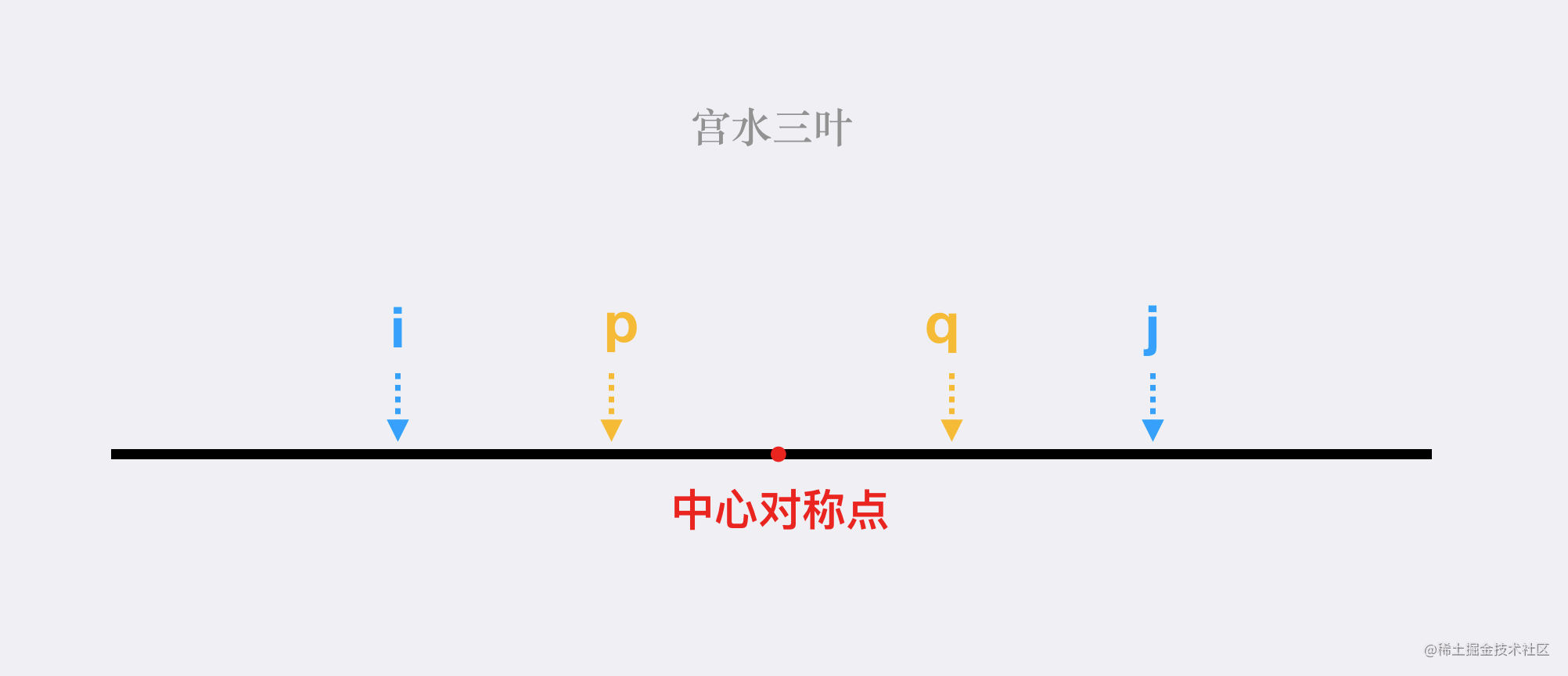# 【每日算法】最大数对和的最小值，贪心解的正确性证明｜Python 主题月

## 题目描述

Tag : 「贪心」

• nums 中每个元素 恰好 在 一个 数对中，且
• 最大数对和 的值 最小 。

输入：nums = [3,5,2,3]

输入：nums = [3,5,4,2,4,6]

• n == nums.length
• 2 <= n <= $10^5$
• n 是 偶数 。
• 1 <= nums[i] <= $10^5$

## 基本分析 & 证明

$(nums, nums[n - 1]), (nums, nums[n - 2]), ... , (nums[(n / 2) - 1], nums[n / 2])$• 调整为 $(nums[i], nums[p])$$(nums[q], nums[j])$：此时最大数对答案为 $nums[q] + nums[j]$，显然 $nums[q] + nums[j] >= nums[i] + nums[j] = ans$。我们要最小化最大数对和，因此该调整方案不会让答案更好；

• 调整为 $(nums[i], nums[q])$$(nums[p], nums[j])$：此时最大数对答案为 $\max(nums[i] + nums[q], nums[p] + nums[j]) = nums[p] + nums[j] >= nums[i] + nums[j] = ans$。我们要最小化最大数对和，因此该调整方案不会让答案更好；

## 贪心

Java 代码：

class Solution {
public int minPairSum(int[] nums) {
Arrays.sort(nums);
int n = nums.length;
int ans = nums + nums[n - 1];
for (int i = 0, j = n - 1; i < j; i++, j--) {
ans = Math.max(ans, nums[i] + nums[j]);
}
return ans;
}
}

Python 3 代码：

class Solution:
def minPairSum(self, nums: List[int]) -> int:
nums.sort()
n = len(nums)
ans = nums + nums[n - 1]
for i in range(1, n//2):
ans = max(ans, nums[i] + nums[n - 1 - i])
return ans

• 时间复杂度：$O(n\log{n})$
• 空间复杂度：$O(\log{n})$